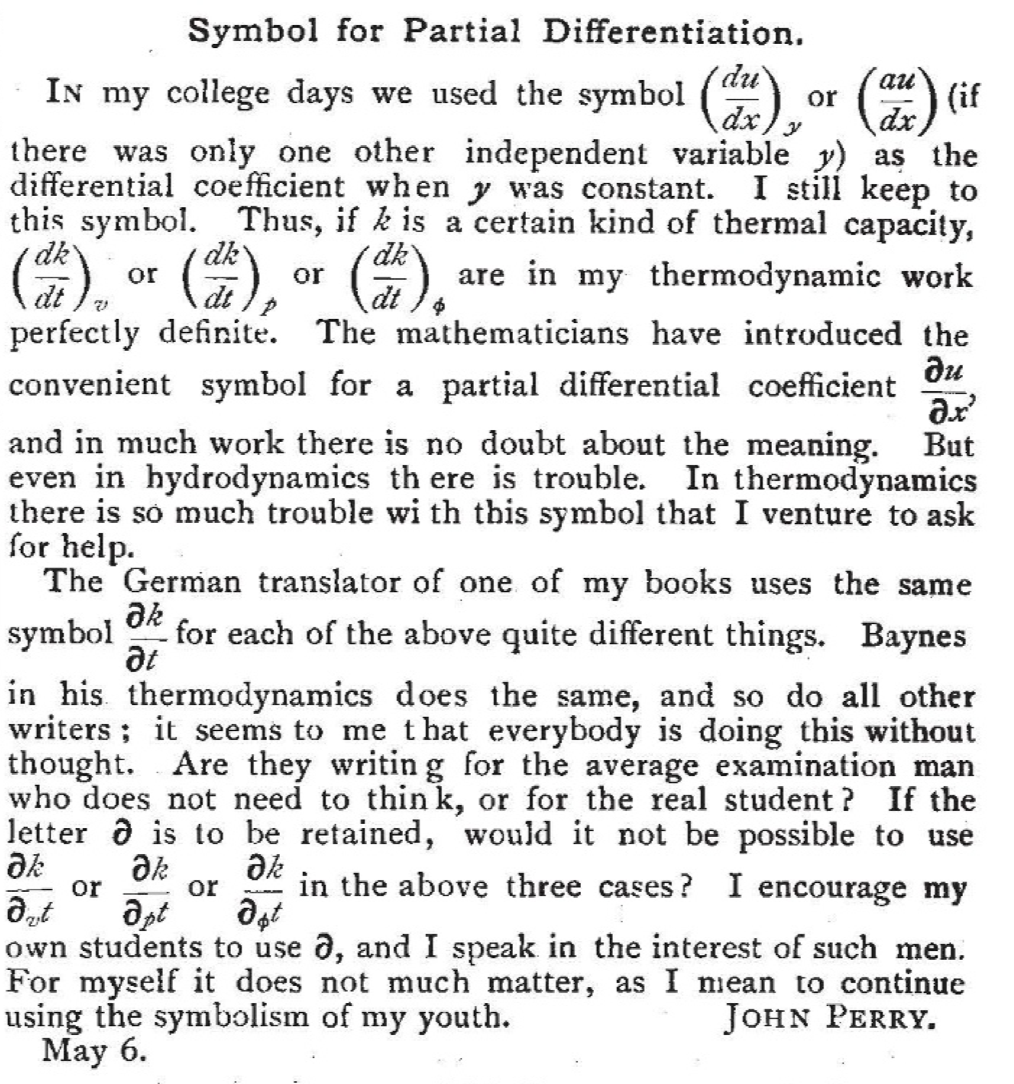# Was Jacobi the first to notice the ambiguity in the partial derivatives notation? And did anyone object to his fix?

In his 1841 article De determinantibus, Jacobi remarked that the notation $$\frac{\partial z}{\partial x}$$ for partial derivatives is ambiguous. He observed that when $$z$$ is a function of $$x,y$$ as well of say $$x,u$$, then the coefficient $$\frac{\partial z}{\partial x}$$ appearing in the linear expansion of $$dz$$ with respect to $$dx,dy$$, denotes something else than the partial derivative $$\frac{\partial z}{\partial x}$$ appearing in the expansion of $$dz$$ wrt $$dx,du$$.

As he himself writes:

In order for the partial differentials, of a function which depends on more than one variable, to be completely determined, it does not suffice to provide the function to be differentiated and the variable with respect to which to differentiate; one must moreover express which quantities remain constant during the differentiation.

A longer excerpt can be found here.

Probably many others have made the same observation since then. Vladimir Arnold for instance makes it in a footnote to his Classical Mechanics. But partial derivatives already existed for 100 years before De determinantibus. (With a slightly different notation that has the same problem.)

Q1: Was Jacobi the first mathematician to point out the ambiguity?

In the same article, Jacobi also proposed a fix for the problem: he suggested we write $$\frac{\partial z(x,y)}{\partial x}$$ for the partial derivative when $$y$$ is held constant, and $$\frac{\partial z(x,u)}{\partial x}$$ when $$u$$ is held constant. This introduces a new problem, since it overloads the notation for function application $$f(x,y)$$, which already had an established and different meaning at that time.

Q2: Did no one object to the suggestion of Jacobi?

Finally, in many physics textbooks (thermodynamics in particular), the problem is fixed by writing $$\left(\frac{\partial z}{\partial x}\right)_y$$ for the derivative of $$z$$ wrt $$x$$ when $$y$$ is held constant.

Q3: Who proposed this fix and when?

• hsm.stackexchange.com seems to be a right forum for such type questions. Jul 17, 2019 at 10:22
• @user64494 hsm has not been able to get out of its beta status in 5 years. And several MO users that could answer the question are not active there. Jul 18, 2019 at 12:10
• There is the same ambiguity in the phrase "other things being equal". (Which other things?) Jul 20, 2019 at 20:37
• Side note - there are actually two ways you can approach it - either be explicit about the variables keeping constant or be explicit about the variables you want to allow to vary. Each have their relative merits. Wrote a short piece on it here: academia.edu/39723296/… Jul 20, 2019 at 21:03
• @johnnyb where could I ask a question or make a comment on your note? Jul 21, 2019 at 8:41

Q2: The German mathematician Paul Stäckel objected to the suggestion of Jacobi: "This notation is ambiguous too, for the symbol is used in two different meanings, in as much as $$f(x, y)$$ is another function of $$x$$ and $$y$$ than is $$f(x, u)$$ of $$x$$ and $$u$$."
Q3: The Irish engineer John Perry advocated the notation $$\left(\frac{dz}{dx}\right)_y$$. I reproduce Perry's 1902 Letter to Nature, where he says he learned this notation at school.